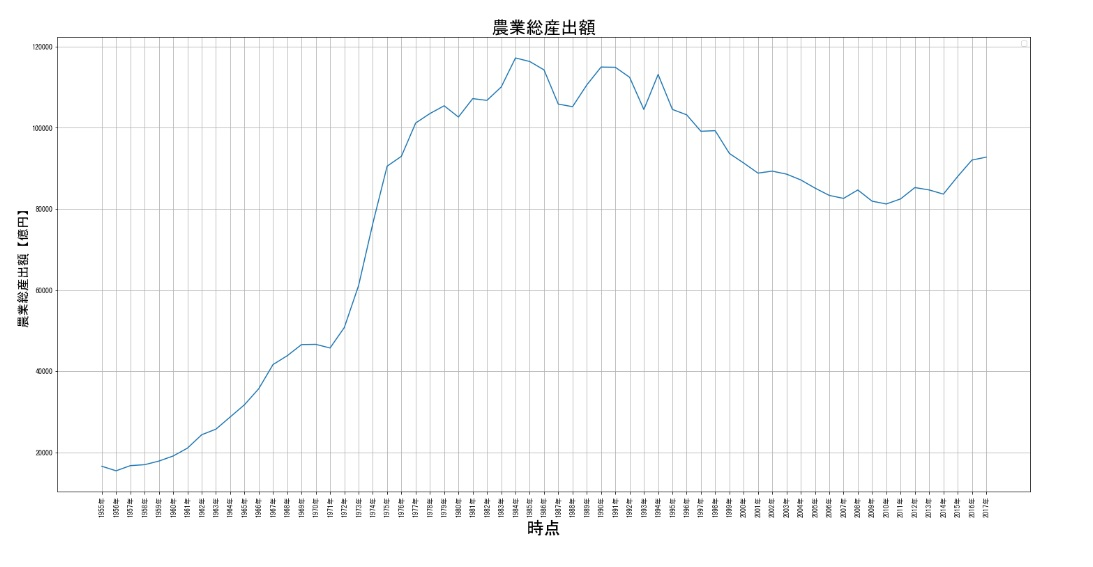# How to correct straight line plot with matplotlib

When I did visualization in matplotlib, the plot was somehow a straight line, so I wrote some notes on how I fixed it.

First, load the data file and display it using Python

I downloaded the data “年次別農業総産出額及び生産農業所得_実額 (Total Agricultural Output and Agricultural Income_Actual Value by Year” from the General Contact Point for Government Statistics as a CSV file.

```# Import the livre required to handle the tabular data
import pandas as pd

# Importing the data
agri_pro_incom = pd.read_csv('Gross agricultural output and agricultural income produced by year_actual.csv', encoding = 'shift-jis', index_col=0)

# Display data
agri_pro_incom```

Executing the above code yields the following DetaFram.However, if you look closely, you will see that the data is in descending order, so you can use sort_index() to change it to ascending order.

Note that sort_index() is in ascending order, but if you want it to be in descending order, use sort_index(ascending=False).

```# Sort the data in ascending order
agri_pro_incom = agri_pro_incom.sort_index()
agri_pro_incom.```Sorting is complete.

## Visualizing data with matplotlib

Now let’s quickly visualize the data using matplotlib.

First, let’s visualize the “Gross Agricultural Output” data as a time series.

```# Import the necessary libraries
from matplotlib import pyplot as plt
import numpy as np

plt.rcParams["figure.figsize"] = (25, 12) # Set plot size
plt.rcParams['font.family'] = "MS Gothic" # Font setting.
plt.xticks(np.range(0, 63)) # Axis ticks from 0 to 63.
plt.xticks(rotation=90) # Flip x-axis labels 90 degrees to avoid covering them
plt.title('Gross agricultural output', fontsize=25)
plt.xlabel('at time', fontsize = 25)
plt.ylabel('Gross agricultural output [billion yen]', fontsize = 18)
plt.legend(['Agricultural output [billion yen]'])
plt.grid()
plt.ticklabel_format(style='plain',axis='y')

# Plot display
plt.plot(agri_pro_incom['Total agricultural output [billions]'])```

The plot obtained when the program is run is shown below.As expected, this was strange, so I investigated various things and found that there was a problem with the data type.

So we use .dtypes to check the DataFrame data type.

```print(agri_pro_incom.dtypes)

Total agricultural output [billion yen] object
Arable_total [billion yen] object
Arable_rice [billion] object
Arable_wheat [billion yen] object
Arable_Minor cereals [billion yen] int64
Cultivated_Beans [billions of yen] object
Arable_potatoes [billions of yen] object
Arable_vegetables_subtotal [billions of yen] object
Arable_vegetables_fruit_crops [billions of yen] object
Cultivated_vegetables_leaf and stem vegetables [100 million yen] object
Arable_vegetables_rootcrops [billion yen] object
Cultivated_Fruits [billion yen] object
Cultivated_Flowers [billion yen] object
Cultivated_Craft crops [billion yen] object
Cultivated_Other crops [¥ billion] object
Livestock_Total [billion yen] object
Livestock_Beef cattle [billion yen] object
Livestock_Dairy cattle [billion yen] object
Livestock_Dairy_cattle_raw_milk [billion yen] object
Livestock_Pig [billion yen] object
Livestock_Poultry [billion yen] object
Livestock_Chicken_eggs_eggs [billion yen] object
Livestock_Chicken_broilers [billion yen] object
Livestock_Sericulture [billion yen] object
Livestock_Other livestock products [billion yen] int64
Processed agricultural products [billion yen] int64
Production agricultural income [billion yen] object
(Reference) Percentage of agricultural income from production to total agricultural output [%] float64
dtype: object```

The data type of each column can be found like this, but when looking at the column you want to visualize, “Gross agricultural output”, it is of type Object.

The Object type is an abstract representation of data, which means that it is treated as a mark rather than a number.

We need to change this to an int type, i.e. an integer type.

Use .astype() to convert the data type and make it visible again.

`agri_pro_incom['Total agricultural output [billion yen]'] = agri_pro_incom['Total agricultural output [billion yen]'].astype(int)`

But the result is again just a straight line.So, after a little more investigation, I found that I needed to remove the ” , (comma)”, so I used .replace() to convert all the numbers to type int.

`agri_pro_incom['Total agricultural output [billion yen]'] = agri_pro_incom['Total agricultural output [billion yen]'].str.replace(','').astype(int)`

Then try the visualisation again.

```from matplotlib import pyplot as plt
import numpy as np
plt.rcParams["figure.figsize"] = (25, 12)
plt.rcParams['font.family'] = "MS Gothic"
plt.xticks(np.arange(0, 63))
plt.xticks(rotation=90)
plt.title('Gross agricultural output', fontsize=25)
plt.xlabel('at time', fontsize = 25)
plt.ylabel('Gross agricultural output [billion yen]', fontsize = 18)
plt.legend(['Agricultural output [billion yen]'])
plt.grid()
plt.ticklabel_format(style='plain',axis='y')
plt.plot(agri_pro_incom['Gross agricultural output [billions]'])```I have successfully completed the plot.